Journal DetailsFormat
Journal
First Published
19 Oct 2012
Publication timeframe
4 times per year
Languages
EnglishOpen Access

# Analysis of Mechanical Behavior of Different Needle Tip Shapes During Puncture of Carbon Fiber Fabric

###### Page range: -
Journal DetailsFormat
Journal
First Published
19 Oct 2012
Publication timeframe
4 times per year
Languages
English

In the present study, the fiber-bending around the needle during the piercing process of the carbon fabric is investigated. In this regard, a mathematical model is established to investigate the bending elongation of the carbon fiber around the needle and the interaction between the carbon fiber and the needle tip. Then the mechanical behavior of the carbon fabric when moving down the tip of the steel needle is analyzed. Based on the performed analysis, a shape curve equation that satisfies the puncture needle tip is established. Furthermore, the influence of different needle tip shapes on the mechanical behavior of the carbon fiber is analyzed. The performance of the needle tip is subjected to different loads, including the puncture template, horizontal tension of the fiber to the needle tip, frictional resistance between the fiber and the needle tip, sliding force, and the bending moment. The performed analysis shows that when the shape of the needle tip assumes the form of curve 10, the downward force, horizontal tension, friction resistance, sliding force, and bending moment are minimized. Accordingly, curve 10 is proposed as the optimal shape for the needle tip. The present study is expected to provide theoretical guidance for selecting overall puncture process parameters.

#### Keywords

Introduction

Recently, integral puncture has been proposed as an innovative processing technique in the production of weaving three-dimensional fabrics. In this technique, laminated carbon fibers are used to produce a woven fabric and then a steel needle array is applied to puncture the fabric integrally. Then, steel needles are replaced one by one with continuous carbon fibers to form the desired puncture fabric. Accordingly, a three-dimensional carbon fiber fabric with a special structure is obtained, which has a promising overall structure and a high fiber volume content. Meanwhile, the produced fabric is an excellent substrate for making high-performance heat-proof and heat-insulating carbon/carbon composite materials. When the tip of the steel needle pierces the carbon fiber fabric, a series of complex movements and effects appear in the fabric; these originate from the puncture action of the steel needle. Analyzing these movements can improve the overall puncture process, optimize the structure of the carbon fiber fabric, and improve the puncture performance.

Studies show that during the puncture process of fabrics, pressure and friction are generated between the needle tip and the carbon fabric. Meanwhile, carbon fabric damage and needle tip damage are the main factors affecting the quality of the punctured fabric [1,2,3]. In this regard, scholars established a mechanical model of the steel needle tip in the top bending mode of the woven fabric, solved the corresponding differential equations subjected to appropriate boundary conditions, and calculated the critical pressure of the tip accordingly [4, 5]. Based on the analysis of the interaction between the needle and the orthogonal laminated woven fabric, the bending elongation and elongation fracture mode of the fiber were proposed and the structural parameters affecting the bending elongation of the fiber were discussed in detail. Moreover, the mechanical model of the steel needle was established and the fiber-bending and elongation mechanisms during the overall puncture process were analyzed [6,7,8,9]. In order to bend the fiber around the needle in the overall puncture process, the puncture needle is deformed by the combination of compression and bending loads originating from the yarn tension. Under these circumstances, the needle tip may undergo displacement under the action of the tension and pierce the fabric as a result. Moreover, the wall contact damages the needle tip consequent to the action of the descending puncture template. In some cases, even the overall puncture may fail because of this phenomenon. In this regard, a mechanical model of the steel puncture needle, which is bent by the fiber, was established [10,11,12,13]. Moreover, the effects of needle punching position and the fabric thickness on the needle resistance are studied empirically. Recently, a numerical simulation based on the concept of virtual fiber has been proposed to establish geometric models of two-dimensional damaged twill fabrics and non-woven fabrics . Furthermore, an explicit dynamic algorithm was proposed to simulate the needle punching process of the fabric cord using the finite element method [15, 16]. Generally, the fiber deflection is analyzed to generate the virtual fiber structure of the needled preform, calculate the effect of the needle punching process on the fiber damage, and improve the mechanical properties of the needle-punched composite material [17, 18]. In the present study, it is intended to explore the fiber movement mode for different positions of the woven fabric. In this regard, different tip shapes and fiber mechanical behavior are analyzed. Finally, the correlation between downforce, horizontal tension, friction resistance, sliding force, bending moment, and needle tip shape is analyzed. The main novelty of the present study lies in its consideration of the interaction between the needle and fibers and optimization of the shape of the needle tip.

Puncture principle

The overall puncture fabric is a three-dimensional fabric that is indirectly formed using the overall puncture technology . Figure 1 shows the schematic puncture process of the carbon fiber fabric. It indicates that the puncture needle tip is subject to downward displacement under the action of the puncture template, so that the equidistant close-packed needles pass through the carbon fiber fabric. During the overall puncture process, needles squeeze the fabric surface, while the fibers may be squeezed, pushed, bent, and deformed; the points A and B denote the bending deformation state of the carbon fiber fabric. Furthermore, the puncture template presses the woven carbon fabric to the bottom end of the puncture needle to prevent the rebound of the woven carbon fabric after puncturing several layers, as the point C. Figure 1 indicates that the overall puncture template should become compact enough to increase the volume content of the overall puncture formed carbon fiber three-dimensional fabric. Meanwhile, the steel needle tip may be shifted and bent under the action of pushing and stretching loads of the fabric fiber. In severe cases, the needle tip loses its main function and it may even break, thereby affecting the smooth implementation of the overall puncture process. Therefore, in addition to studying the bending and elongation mechanism of the fiber in the overall puncture process, it is essential to analyze interactions between the fiber and the needle tip. Then the corresponding mechanical model can be established to reduce the stress of the fiber on the needle originating from the penetration of the woven carbon cloth. In this regard, optimizing the shape of the needle tip is an important research issue of the overall puncture technology.

Shape analysis of the tip

The puncture needle is composed of a steel needle shaft and a steel needle tip. The needle tip may have different shapes. In the present study, cone shaped needles made by multi-station mechanical grinding are utilized. It is worth noting that the curve of the cone surface should be set before grinding. Figure 2 presents an enlarged schematic diagram of the needle and the needle tip. Let the vertex of the needle tip be the coordinate origin O, and the direction of the needle bar is the positive direction of the y-axis. Moreover, rn and Ln denote the radius of the needle shaft and the length of the needle tip, respectively.

In order to prevent complex calculations, it is essential to ensure that the shape of the needle tip is not too complicated. In this regard, a simple tip curve equation in the following form is considered: $y=a0⋅xm$y = {a_0} \cdot {x^m} where m > 0, x ∈ [0, rn], y ∈ [0, − Ln]. In the present study, a0 is set to $a0=−Ln/rnm${a_0} = - {L_n}/r_n^m . Moreover, five different values, including 0.5, 0.7, 1.0, 1.5, and 2.0 are considered for m. Accordingly, five tip shape curves referred to as y1 (curve 1), y2 (curve 2), y3 (curve 3), y4 (curve 4), and y5 (curve 5) are defined. The needle bar radius and the needle tip length are rn = 0.6 mm and Ln = 10 mm , respectively. Different tip shapes are illustrated in Figure 3a.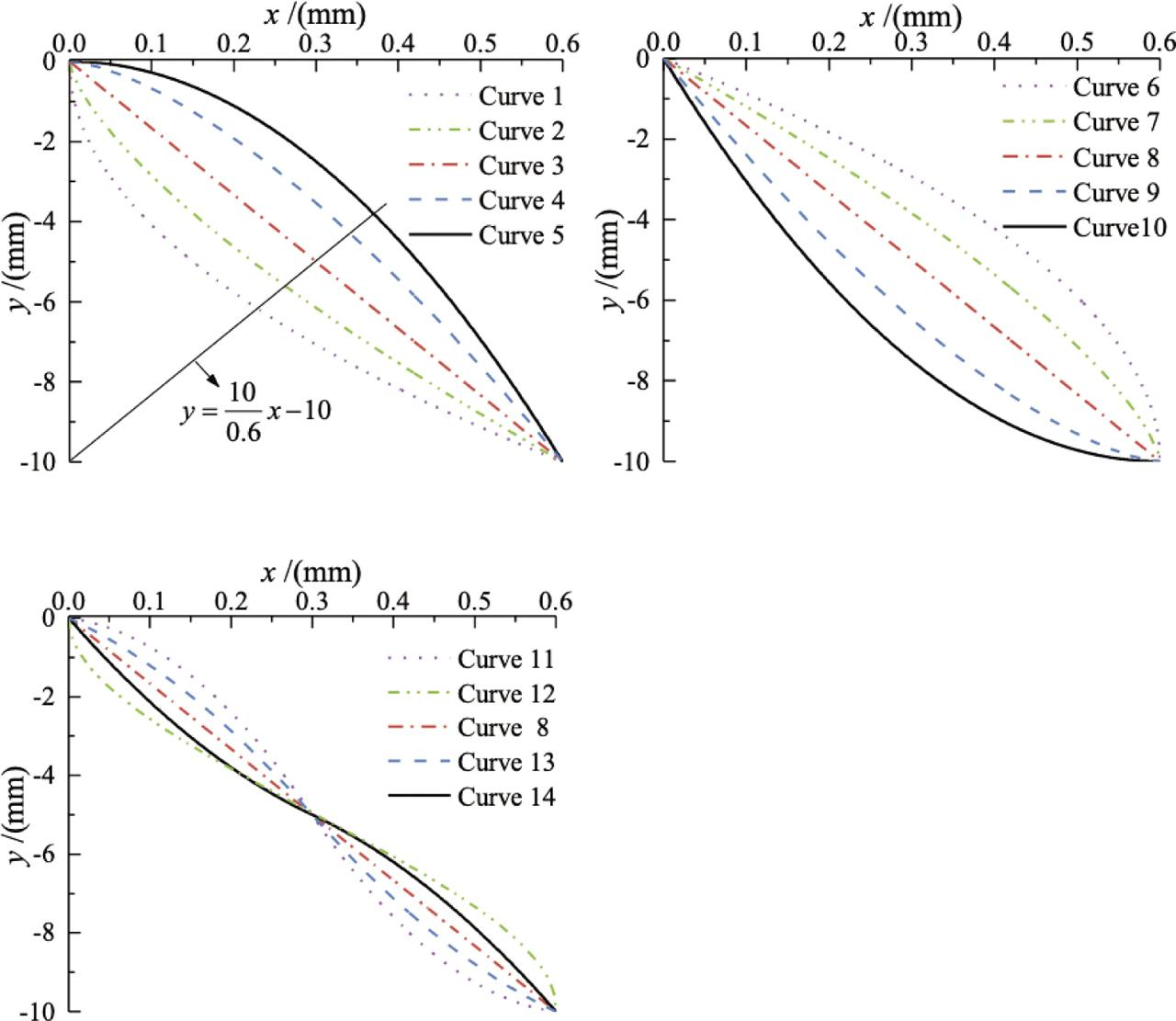Shape curves of the tip. (a) Needle tip shape curves 1–-5, (b) Needle tip shape curves 6–-10 and. (c) Structured tip shape curves.

When the five curves in Figure 3a are subject to clockwise rotation by 180° along the line $y=100.6x−10$y = {{10} \over {0.6}}x - 10 , this leads to the formation of five additional curves. The corresponding tip shape curves can be expressed in the following form: $y=Lnrnm⋅(rn−x)m−Ln$y = {{{L_n}} \over {r_n^m}} \cdot {\left( {{r_n} - x} \right)^m} - {L_n} where m can be 0.5, 0.7, 1.0, 1.5, and 2.0, and the corresponding shape curves of the needle tip are referred to as y6 (curve 6), y7 (curve 7), y8 (curve 8), y9 (curve 9), and y10 (curve 10), respectively. It is worth noting that y3 = y8.

Studies  show that when the shape curve of the needle tip is y3, the best mechanical properties can be achieved. Therefore, other curves near the straight-line y3 should be found for analysis. In this regard, a curve y11 (curve 11) is considered around the straight-line. This curve can be expressed as y11 = ax3 + bx2 + cx + d, and we set the two points on the curve as (x0, y0) and (x1, y1). This curve is subjected to the following boundary conditions: ${x0=0y0=0$\left\{ {\matrix{ {{x_0} = 0} \hfill \cr {{y_0} = 0} \hfill \cr } } \right.${x1=rny1=−Ln$\left\{ {\matrix{ {{x_1} = {r_n}} \cr {{y_1} = - {L_n}} \cr } } \right.${f′(x0,y0)=0f′(x1,y1)=1$\left\{ {\matrix{ {f'\left( {{x_0},{y_0}} \right) = 0} \cr {f'\left( {{x_1},{y_1}} \right) = 1} \cr } } \right.

Based on these boundary conditions, parameters can be calculated as a = 95.37, b = −85, c = 0, and d = 0. Consequently, the curve equation can be expressed in the form stated below: $y11=95.37x3−85x2${y_{11}} = 95.37{x^3} - 85{x^2}

Now the needle tip shape curve y12 (curve 12) can be formed around the line. Figure 4 illustrates the tip shape y12 = f1(x,y), where point Q is on the line TV, line QT is perpendicular to line OO1, and the coordinates of point T is (0.15, −2.5). Moreover, the coordinates of point T1 is (0.45, −7.5), the coordinate of point O is (x0, y0), the coordinate of point V is (x, y), the coordinate of point R is (xR, yR), and the coordinate of point Q is (xQ, yQ); then: ${(x0−xQ)2+(y0−yQ)2=LQO2(xR−xQ)2+(yR−yQ)2=LQO2$\left\{ {\matrix{ {{{\left( {{x_0} - {x_Q}} \right)}^2} + {{\left( {{y_0} - {y_Q}} \right)}^2} = L_{QO}^2} \cr {{{\left( {{x_R} - {x_Q}} \right)}^2} + {{\left( {{y_R} - {y_Q}} \right)}^2} = L_{QO}^2} \cr } } \right.

The coordinates of points O and R are (0,0) and (0.3, −5.0), respectively. Assuming LQO = 50, then the coordinates (xQ, yQ) can be obtained.

The equation of the curve OR is: $(x−xQ)2+(y12−yQ)2=LQO2${\left( {x - {x_Q}} \right)^2} + {\left( {{y_{12}} - {y_Q}} \right)^2} = L_{QO}^2

Similarly, the curve RO1 can be calculated. Consequently, y12 can be expressed in the form stated below: $y12={−2515.27−(x−50.15)2+0.5 x∈[0,0.3]2515.27−(x+49.55)2−10.5x∈(0.3, 0.6]${y_{12}} = \left\{ {\matrix{ { - \sqrt {2515.27 - {{\left( {x - 50.15} \right)}^2}} + 0.5\,} & {x \in \left[ {0,0.3} \right]} \cr {\sqrt {2515.27 - {{\left( {x + 49.55} \right)}^2}} - 10.5} & {x \in (0.3,\,0.6]} \cr } } \right.

When LQO = 100, y13 (curve 13) can be obtained in the same way. In this case, the curve equation can be written in the form stated below: $y13={−10042.27−(x−100.15)2+3.5 x∈[0,0.3]10042.27−(x+99.55)2−13.5x∈(0.3, 0.6]${y_{13}} = \left\{ {\matrix{ { - \sqrt {10042.27 - {{\left( {x - 100.15} \right)}^2}} + 3.5\,} & {x \in \left[ {0,0.3} \right]} \cr {\sqrt {10042.27 - {{\left( {x + 99.55} \right)}^2}} - 13.5} & {x \in (0.3,\,0.6]} \cr } } \right.

Since curve 13 along curve 3 is symmetric, y14 (curve 14) can be obtained and the corresponding curve equation can be expressed in the form stated below: $y14={10042.27−(x+99.85)2−8.5 x∈[0,0.3]−10042.27−(x−100.45)2−1.5x∈(0.3, 0.6]${y_{14}} = \left\{ {\matrix{ {\sqrt {10042.27 - {{\left( {x + 99.85} \right)}^2}} - 8.5\,} & {x \in \left[ {0,0.3} \right]} \cr {-\sqrt {10042.27 - {{\left( {x - 100.45} \right)}^2}} - 1.5} & {x \in (0.3,\,0.6]} \cr } } \right.

Figure 3c shows the shape curves of structured steel needle tip.

Analysis of needle tip force

During the downward movement of the puncture template, the needle passing through the woven carbon fabric gradually moves from the needle tip to the needle shaft. Under the action of the needle tip cone of the steel needle, the fibers in the woven carbon cloth produce a series of complicated changes such as displacement, pushing, bending, and elongation. Moreover, they interact with the steel needle to cause the steel needle to press down and bend. In the process of the fiber slipping on the tip of the steel needle, 1,001 contact points of fiber and needle tip curve are selected as the research objects, which are represented as P0, P1, P2, …, Pn. $Pn=(xn,yn)${P_n} = \left( {{x_n},{y_n}} \right) where: xn = n·rn/1,000, and n = 0, 1, 2,…, 1,000.

Figure 5 shows that the middle point Pk is considered as an example to analyze the force of the needle tip. First, the tip of the steel needle is subjected to the horizontal tension caused by the elongation of the fiber, which is recorded as FLK. It is worth noting that the tension FLK is decomposed into normal component force FL1K and tangential component force FL2K. Second, when the puncture template presses down the woven carbon cloth, the tip of the steel needle is subjected to the downward force generated by the carbon fiber fabric. Since the puncture template moves at a constant speed during the puncture process, the downward force at each point where the carbon fiber contacts the needle tip is different, and this is recorded as FNK. It should be indicated that the downward force FNK is decomposed into normal component force FN1K and tangential component force FN2K.

Fiber tension between adjacent steel needles

Fiber-bending has many forms. In Figure 6, the length ⌒B1kB2k of the fiber-bending around the needle between adjacent steel needles is analyzed. Figure 6a and b illustrates that fiber elongation includes horizontal elongation and vertical elongation. The dotted line between C2KB′2k in Figure 6a is the projection of ⌒C2kB2k on the plane in Figure 6b.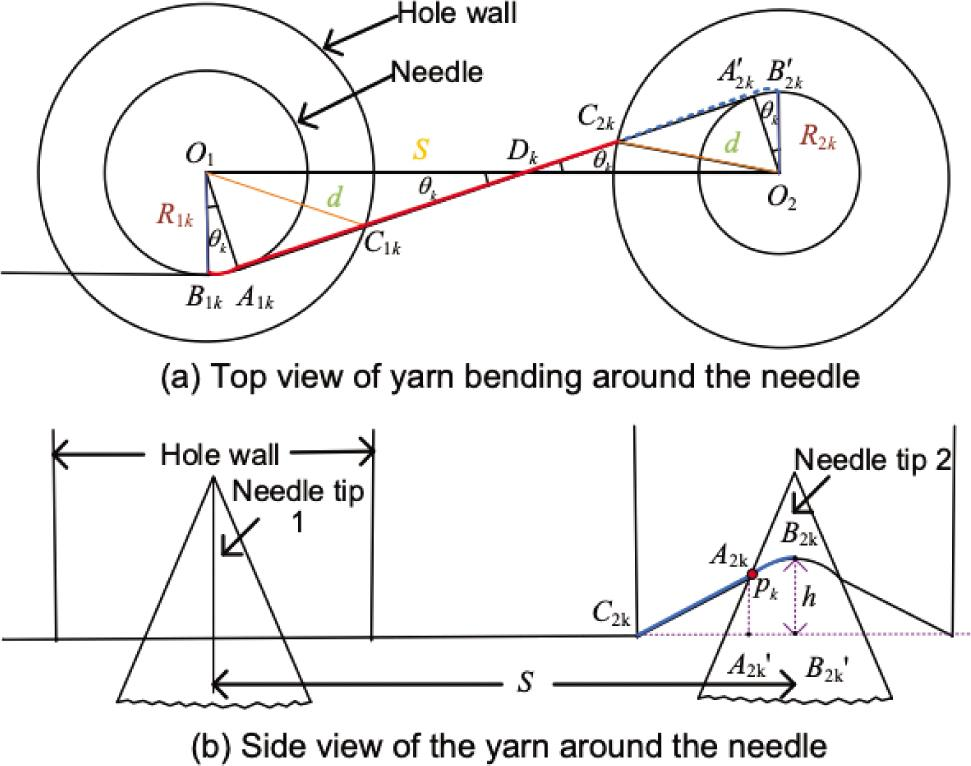Drawing model of the bending fiber around the needle. (a). Top view of yarn bending around the needle and. (b). Side view of the yarn around the needle.

The fiber length ⌒B1kB2k after bending and stretching at point Pk between two adjacent steel needles is mathematically expressed as the following: $⌢,B1kB2k=⌢,B1kA1k+A1kDk+DkC2k+⌢, C2kB2k$^\frown,{B_{1k}}{B_{2k}} = ^\frown,{B_{1k}}{A_{1k}} + {A_{1k}}{D_k} + {D_k}{C_{2k}} + ^\frown,\,{C_{2k}}{B_{2k}}

Eqs (14)–(17) give the four lengths of radians as ⌒B1kB2k. These equations are derived from the geometric correlation in Figure 6: $⌢,B1kA1k=R1kθk=R1k arcsin(R1k+R2k)/S$^\frown,{B_{1k}}{A_{1k}} = {R_{1k}}{\theta _k} = {R_{1k}}\,{arcsin}\left( {{R_{1k}} + {R_{2k}}} \right)/S where R1k, R2k, and S denote the radius of the needle tip of the contact point between the fabric and the needle tip 1, the radius of the needle tip of the contact point between the fabric and the needle tip 2, and the center distance between two adjacent holes of the puncture template, respectively. $A1kDk=O1Dk2−O1A1k2=[SR1k/(R1k+R2k)]2−R1k2${A_{1k}}{D_k} = \sqrt {{O_1}D_k^2 - {O_1}A_{1k}^2} = \sqrt {{{\left[ {S{R_{1k}}/\left( {{R_{1k}} + {R_{2k}}} \right)} \right]}^2} - R_{1k}^2} $DkC2k=DkA2k′−C2kA2k′=[SR2k/(R1k+R2k)]2−R2k2−dh2−R2k2${D_k}{C_{2k}} = {D_k}A_{2k}^\prime - {C_{2k}}A_{2k}^\prime = \sqrt {{{\left[ {S{R_{2k}}/\left( {{R_{1k}} + {R_{2k}}} \right)} \right]}^2} - R_{2k}^2} - \sqrt {d_h^2 - R_{2k}^2} where dh is the diameter of the hole. Further, $⌢,C2kB2k=[B2kB2k′2+(C2kA2k′+A2k′B2k′)2]=[h2+(dh2−R2k2+R2karcsinR1k+R2ks)2]$\matrix{ {^\frown,{{\rm{C}}_{2{\rm{k}}}}{{\rm{B}}_{2{\rm{k}}}} = \sqrt {\left[ {{B_{2k}}B_{2k}^{\prime 2} + {{\left( {{C_{2k}}A_{2k}^\prime + A_{2k}^\prime B_{2k}^\prime} \right)}^2}} \right]} } \hfill \cr { = \sqrt {\left[ {{h^2} + {{\left( {\sqrt {d_h^2 - R_{2k}^2} + {R_{2k}}\,{arcsin}{{{R_{1k}} + {R_{2k}}} \over s}} \right)}^2}} \right]} } \hfill \cr } where h is the distance from the fabric to the piercing template at any time.

According to the theory of fiber material mechanics, the tension FLk generated by bending and elongating fiber is expressed as the following : $FLk=EcAzεk${F_{Lk}} = {E_c}{A_z}{\varepsilon _k} where Ec, Az, and ɛk denote the elastic modulus of the fiber, the cross-sectional area of the carbon cloth fiber bundle, and the fiber elongation, respectively. Meanwhile, the elongation ɛ is presented as follows: $εk=(B1kB2k−2rn)/(2rn)${\varepsilon _k} = \left( {{B_{1k}}{B_{2k}} - 2{r_n}} \right)/\left( {2{r_n}} \right)

Then, the fiber normal-tension FL1k and fiber tangential tension FL2k are obtained, which are described as the following: ${FL1k=FLk⋅cosθkFL2k=FLk⋅sinθk$\left\{ {\matrix{ {{F_{L1k}} = {F_{Lk}} \cdot \cos {\theta _k}} \hfill \cr {{F_{L2k}} = {F_{Lk}} \cdot \sin {\theta _k}} \hfill \cr } } \right.

Downward force, frictional resistance, and sliding force

When the downward force of the carbon fiber fabric on the needle tip at the point Pk is FNk, the normal pressure FN1k and the tangential pressure FN2k can be obtained: ${FN1k=FN⋅sinθkFN2k=FN⋅cosθk$\left\{ {\matrix{ {{F_{N1k}} = {F_N} \cdot \sin {\theta _k}} \hfill \cr {{F_{N2k}} = {F_N} \cdot \cos {\theta _k}} \hfill \cr } } \right.

Thus, the frictional resistance calculation equation of the intermediate point Pk is established: $FZk=(FL1k+FN1k)⋅ρ${F_{Zk}} = \left( {{F_{L1k}} + {F_{N1k}}} \right) \cdot \rho where ρ is the friction coefficient between the fiber and the steel needle.

Then, the total frictional resistance FZ suffered by the tip of the steel needle is stated as follows: $FZ=[(FL11+FN11)+(FL12+FN12)+…+(FL1n+FN1n)]⋅ρ${F_Z} = \left[ {\left( {{F_{L11}} + {F_{N11}}} \right) + \left( {{F_{L12}} + {F_{N12}}} \right) + \ldots + \left( {{F_{L1n}} + {F_{N1n}}} \right)} \right] \cdot \rho

Moreover, the equation for calculating the sliding force FHk at the intermediate point Pk is expressed as follows: $FHk=FN2k−FL2k${F_{Hk}} = {F_{N2k}} - {F_{L2k}}

Then, the total sliding force FH experienced by the tip of the steel needle is: $FH=[(FN21−FL21)+(FN22−FL22)+…+(FN2n−FL2n)]${F_H} = \left[ {\left( {{F_{N21}} - {F_{L21}}} \right) + \left( {{F_{N22}} - {F_{L22}}} \right) + \ldots + \left( {{F_{N2n}} - {F_{L2n}}} \right)} \right]

Bending moment

Figure 7 shows the horizontal tension FLk of the steel needle tip at point Pk and the additional clockwise moment Mk of the needle tip at this point under this force, where: Mk = − FLkyk.

From the force analysis of the tip of the steel needle, we obtain the total bending moment M produced by the horizontal tension FLk of the steel needle. $M=M1+M2+…+Mn$M = {M_1} + {M_2} + \ldots + {M_n}

Results and discussion

In this section, from Table 1 and Eqs (12)–(26), the downward force FN, horizontal tension FL, friction force FZ, sliding force FH, and bending moment M for the abovementioned 14 different needle tip shapes are calculated.

Force solution parameters .

ParameterValueUnitNote
Ec2.30*1011PaModulus of elasticity
Dc9.00*10−4mmFiber diameter
S2.30mmDistance between holes
dh2.00mmHole diameter
H0.50mmFiber Z-direction elongation
P0.80Coefficient of friction

It is ensured that the puncture template moves at the same speed under different needle tip shapes and keeps a constant motion state at all times; accordingly, Figures 8 and 9 show the numerical change curve of the downforce FN corresponding to different needle tip shape curves and the numerical change curve of horizontal tension FL, respectively. Moreover, Figures 10 and 11 illustrate the numerical curve of the friction resistance FZ corresponding to different needle tip shapes and the numerical curve of sliding force FH, respectively. Figure 12 shows the stress distribution diagram of the bending moment at different positions of the needle tip, which determines the position of the dangerous point of the needle tip section of different steel needles.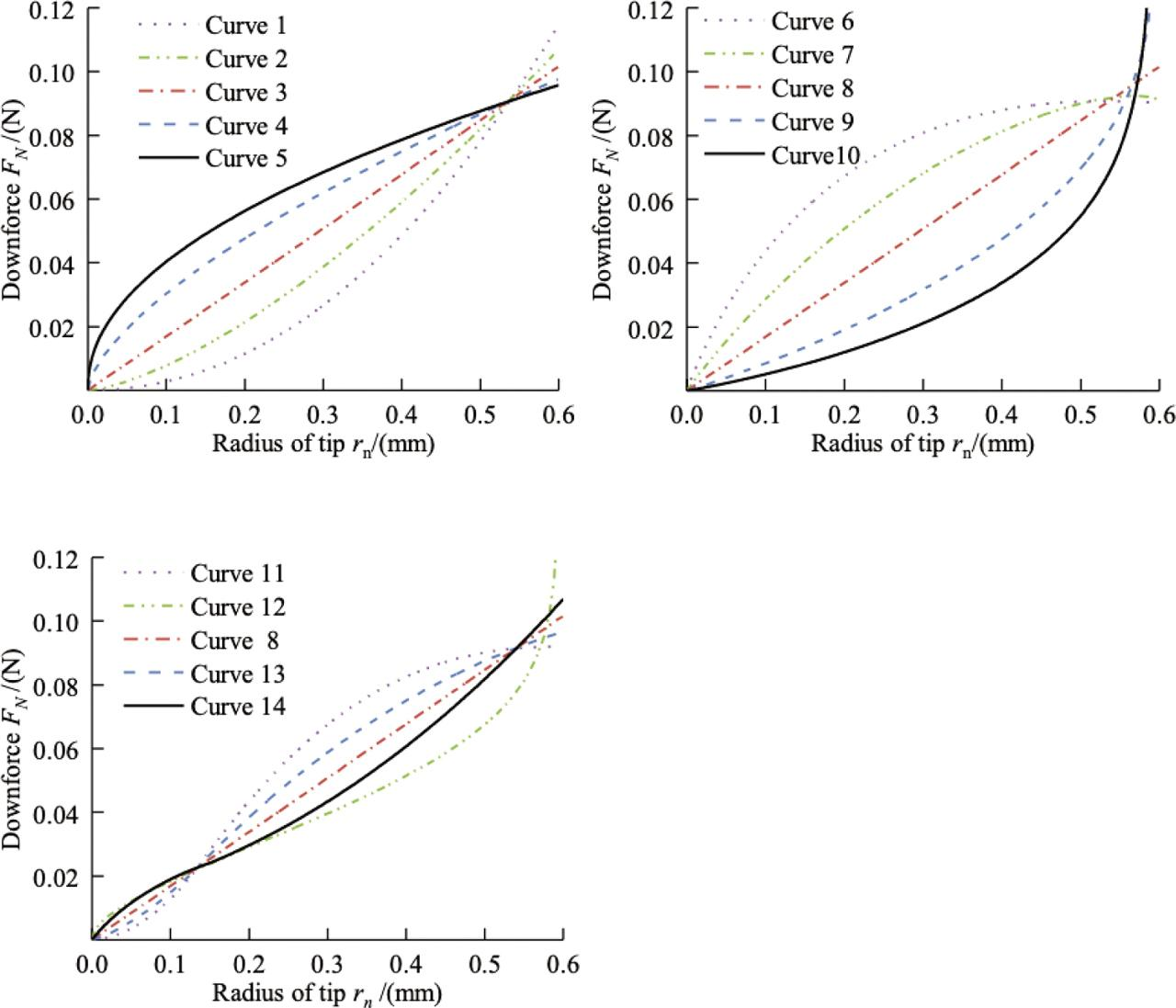Downward force FN corresponding to different needle tip curves. (a) Downward force of needle tip curves 1—5; (b) Downward force of needle tip curves 6—10; and. (c) Downforce of the constructed needle tip curves.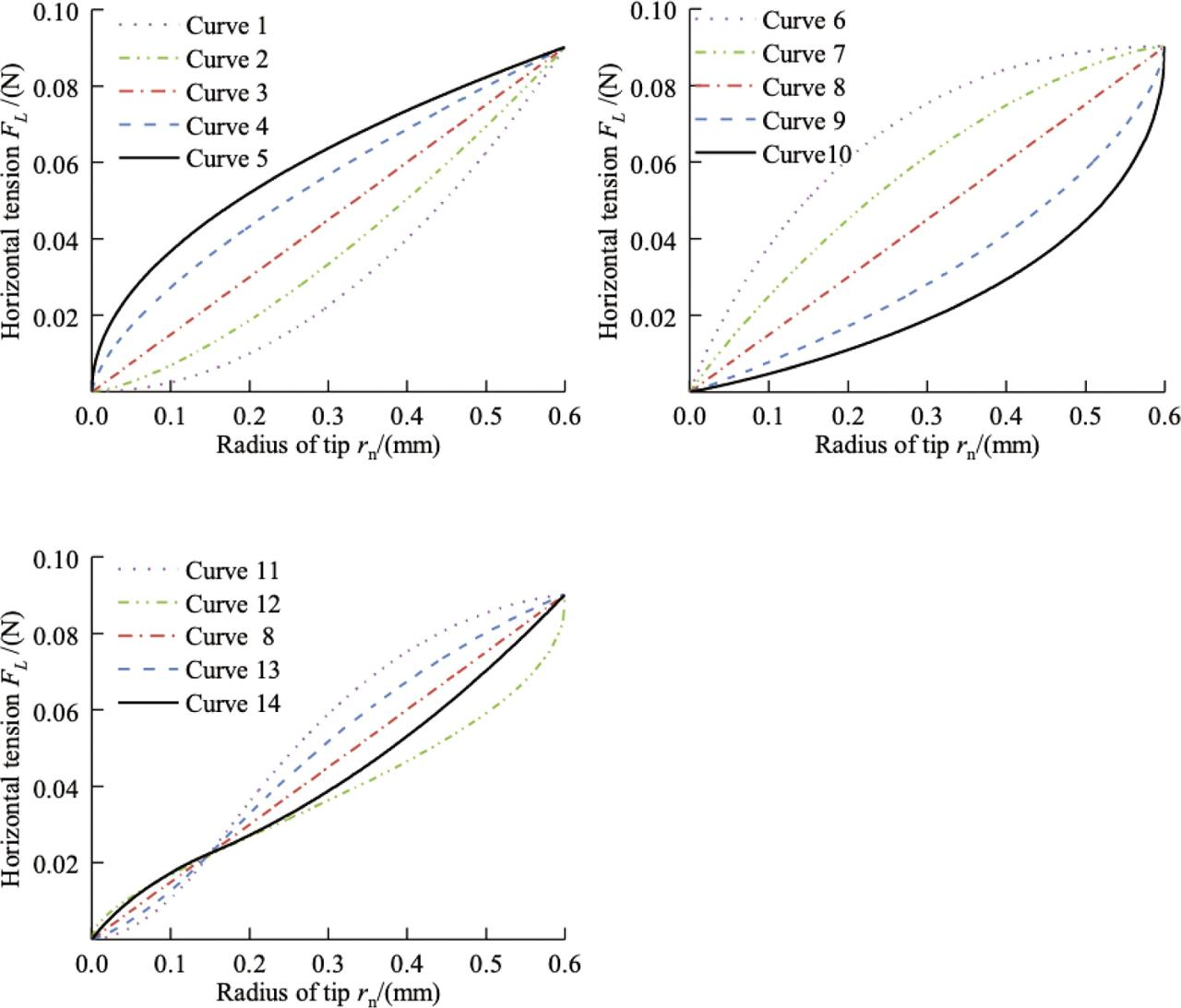Horizontal tension FL corresponding to different needle tip curves. (a) Horizontal tension of needle tip curves 1—5; (b) Horizontal tension of needle tip curves 6—10; and. (c) Horizontal tension of the constructed needle tip curves.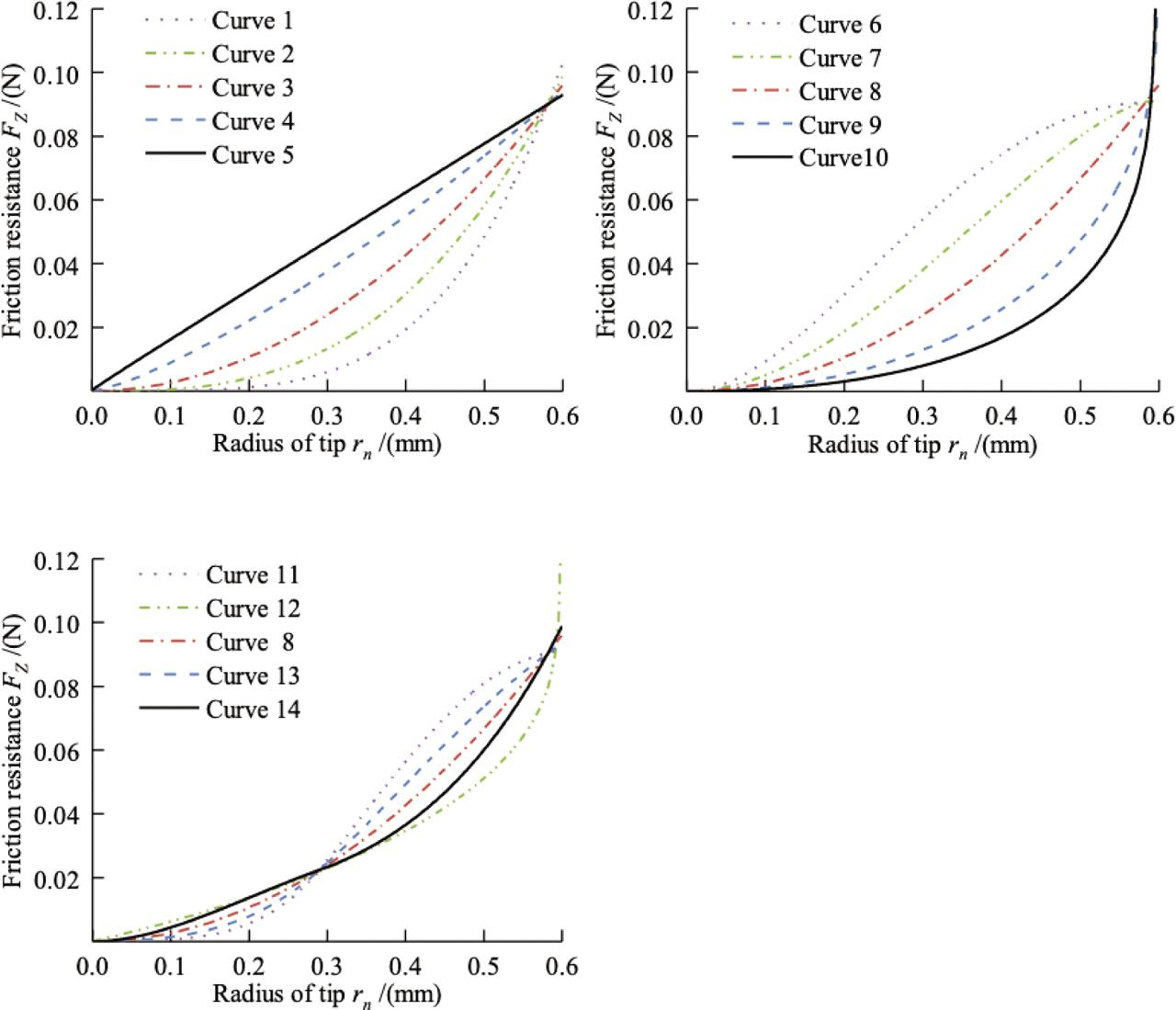Friction resistance FZ corresponding to different needle tip curves. (a) Friction resistance of the needle tip curves 1—5; (b) Friction resistance of the needle tip curves 6—10; and. (c) Frictional resistance of the constructed needle tip curves.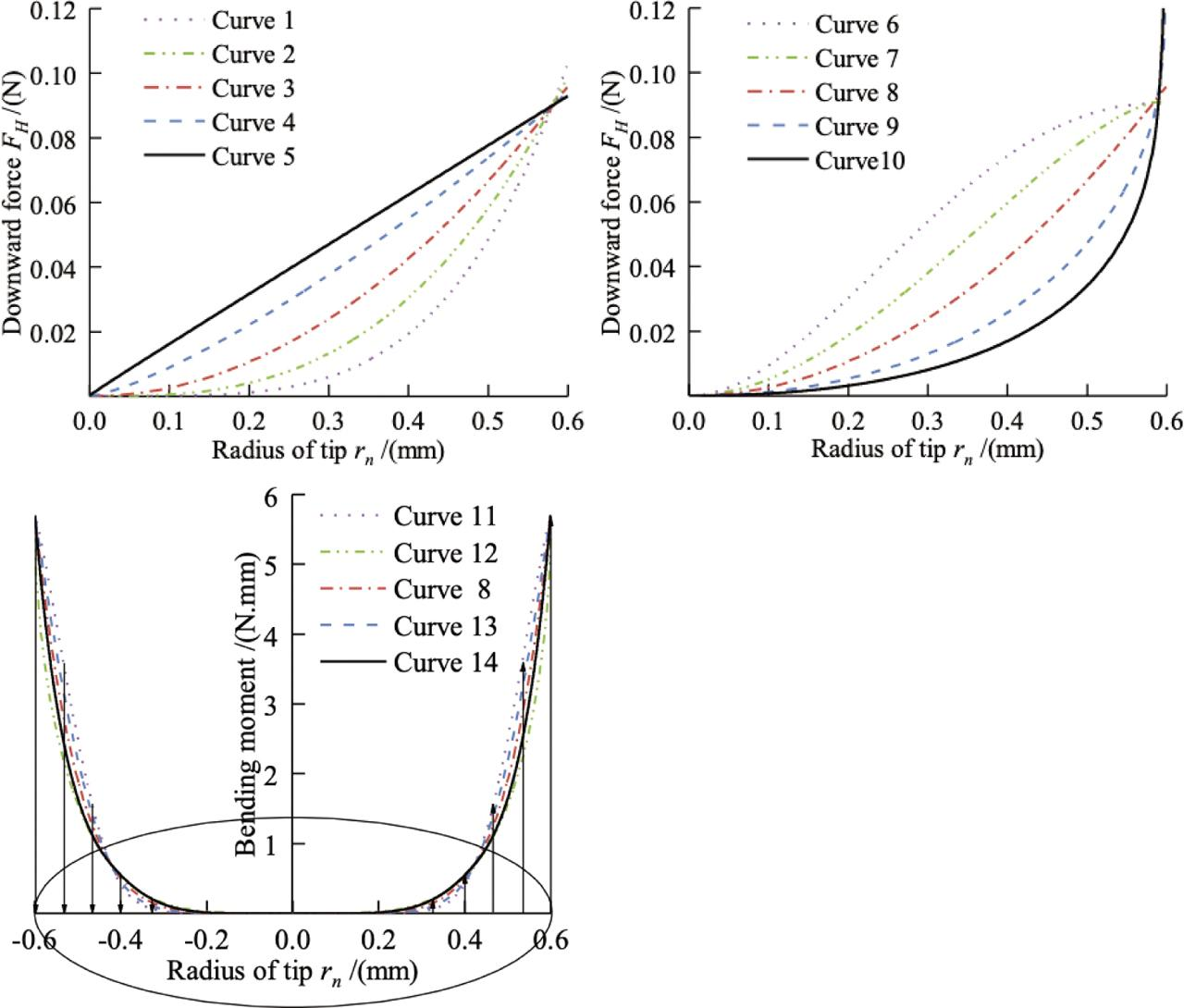The sliding force FH corresponding to different needle tip curves. (a) Downward force of the needle tip curves 1—5; (b) Downward force of the needle tip curves 6–−10; and. (c) The sliding force of the constructed needle tip curves.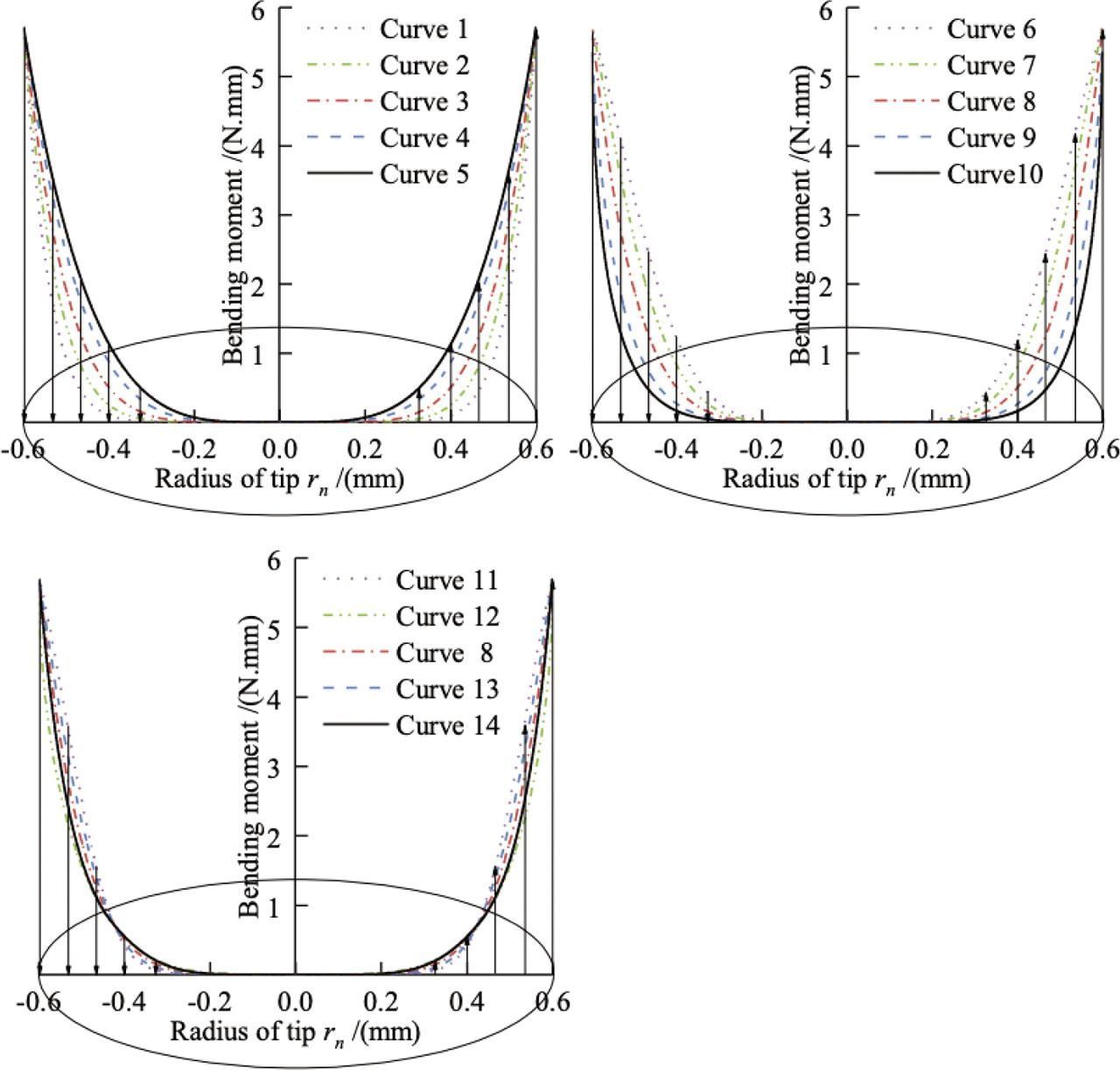Tip bending moment stress diagram. (a) Bending moment of tip curves 1—5; (b) Bending moment of tip curves 6—10; and. (c) Bending moment of the constructed tip curves.

Moreover, Table 2 presents the sum of downward force FN, horizontal tension FL, friction force FZ, sliding force FH, and the bending moment M corresponding to different needle tip shape curves.

Sum of force values.

Needle tip shapeFN/(N)FL/(N)FZ/(N)FH/(N)M/ (N·mm)
Curve 1139.59114.13127.52127.00950.72
Curve 2172.82148.04160.91160.381,175.15
Curve 3214.74190.43202.95202.411,427.68
Curve 4268.95244.94257.23256.691,713.95
Curve 5309.77285.82298.07297.531,904.83
Curve 6329.14304.95317.38316.852,095.94
Curve 7273.17249.06261.43260.891,789.54
Curve 8214.74190.43202.95202.411,427.68
Curve 9157.11130.46144.71144.211,018.73
Curve 10121.6995.04109.61109.16759.85
Curve 11238.26213.73226.42225.891,643.87
Curve 12192.35167.90181.39179.431,198.52
Curve 13225.57201.25213.78213.241,531.67
Curve 14205.75181.27193.92193.391,332.01

It is observed that the downward force, horizontal tension, friction resistance, sliding force, and bending moment at 1,001 points where the needle tip contacts the fiber corresponding to different needle tip shape curves vary with the diameter of the needle tip. It is worth noting that the downforce, horizontal tension, friction resistance, sliding force, and bending moment values of curve 10 are lower than those of other needle tip shape curves. When the needle tip shape corresponds to curve 10, in other words when the curve equation is $y10=100.62.0⋅(0.6−x)2.0−10${y_{10}} = {{10} \over {{{0.6}^{2.0}}}} \cdot {\left( {0.6 - x} \right)^{2.0}} - 10 , the downward pressure FN of the puncture template to the needle tip and the horizontal tension FL of the fiber to the needle tip are the lowest, which equal to121.69 N and 95.04 N, respectively. However, when the needle tip shape corresponds to curve 6, in other words when the curve equation is $y6=100.60.5⋅(0.6−x)0.5−10${y_6} = {{10} \over {{{0.6}^{0.5}}}} \cdot {\left( {0.6 - x} \right)^{0.5}} - 10 , the downward pressure of the puncture template on the needle tip and the horizontal tension of the fiber to the needle tip are the highest, which are 329.14 N and 304.95 N, respectively.

Further analysis shows that when the tip shape curve corresponds to curve 10, the total friction resistance between the fiber and the tip is 109.61 N, and the total sliding force between the fiber and the steel needle is the lowest, which is 109.16 N. Moreover, when the shape curve of the steel needle tip assumes the form of curve 6, the total friction resistance between the fiber and the needle tip and the total sliding force between the fiber and the needle tip are the highest, which equal 317.38 N and 316.85 N, respectively. It is observed that when curve 10 is selected for the needle tip shape, the friction and sliding force between the fiber and the needle tip are the smallest. This indicates that when the needle tip shape is curve 10, the movement between the fiber and the needle tip is optimal among the abovementioned different needle tip shapes. Meanwhile, when the needle tip shape is curve 6, the movement between the fiber and the needle tip is the worst. Moreover, it is found that the frictional force is the same as the sliding force, which is consistent with the condition that the downward pressure of the puncture template should always be kept always uniform. Therefore, the correctness of the established mechanical model is verified.

When the needle tip shape assumes the form of curve 10, the bending moment that the needle tip can withstand is the smallest, which is 759.85 N·mm. This shows that the shape of this tip is the one that is the most optimal. When the needle tip shape corresponds to curve 1, the bending moment that the needle tip can withstand and the downward pressure of the puncture template on the needle tip are 950.72 N.mm and 139.59 N, respectively. Moreover, the horizontal tension of the fiber to the needle tip, the frictional resistance between the fiber and the needle tip, and the sliding force between the fiber and the needle tip are 114.13 N, 127.52 N, and 127.00 N, respectively. When the needle tip shape corresponds to curve 2, the bending moment that the needle tip can withstand and the downward pressure of the puncture template on the needle tip are 1,175.15 N.mm and 172.82 N, respectively. Moreover, the horizontal tension of the fiber to the needle tip, the frictional resistance between the fiber and the needle tip, and the sliding force between the fiber and the needle tip are 148.04 N, 160.91 N, and 160.38 N, respectively. When the needle tip shape corresponds to curve 9, the bending moment that the needle tip can withstand and the downward force are 1,018.73 N·mm and 157.11 N, respectively. Moreover, the horizontal tension, the friction resistance, and the sliding force are 130.46 N, 144.71 N, and 144.21 N, respectively. When the tip shape corresponds to curve 12, the bending moment that the tip can withstand and the downward force are 1,198.52 N. mm and 192.35 N, respectively. Moreover, the horizontal tension, the friction resistance, and the sliding force are 167.90 N, 181.39 N, and179.43 N, respectively. The abovementioned data shows out of these four types, the force of the needle tip under the shape is better. The present study provides theoretical guidance for the selection of overall puncture process parameters and the selection of the needle tip shape.

In order to ensure the smooth progress of the overall puncture process and meet the displacement requirements of the needle tip without having any contact with the hole wall of the puncture template, the displacement of the needle tip is determined under the action of fiber tension. It is found that when the steel needle punctures the fabric once, the requirements of the puncture system for its displacement is met . Accordingly, different parameters, including the down force of the needle tip by the puncture template, horizontal tension of the fiber to the needle tip, frictional resistance between the fiber and the needle tip, sliding force between the fiber and the needle tip, and the numerical curve of the bending moment of the needle tip under horizontal tension, are considered and analyzed in this article. Then, a comparative analysis is carried out. Based on the obtained results, quadratic curve is proposed as the optimal shape for the needle tip.

CONCLUSIONS

In the present study, based on the analysis of the principle of the puncture of the carbon fiber fabric by the needle tip and to ensure the smooth progress of the overall puncture process, 14 curve equations satisfying the shape of the puncture steel needle tip are selected. Moreover, 14 kinds of needle tips under the action of the puncture template and fiber tension are analyzed. The needle tip is subjected to the downward pressure of the puncture template, the horizontal tension of the fiber to the needle tip, the friction resistance between the fiber and the needle tip, the sliding force between the fiber and the needle tip, and the needle tip bending moment under horizontal tension. Furthermore, the fiber movement mode, mechanical model, and solving of equations of the woven fabric at different needle tip positions are established, and different needle tip shapes and fiber mechanical behavior are analyzed.

Based on the different needle tip shape curves, force analysis and solving of equations are carried out and the numerical curves of the downward force of the needle tip subjected to the puncture template; the horizontal tension of the fiber to the needle tip; the frictional resistance between the fiber, the needle tip, and the fiber; and the sliding force between the needle tip and the bending moment of the needle tip under horizontal tension are analyzed. The analysis shows that when the shape of the needle tip curve corresponds to curve 10, the downward force, horizontal tension, friction resistance, sliding force, and bending moment are the smallest. Therefore, the shape of the needle tip is optimal when curve 10 is applied. It should be indicated that curve 1, curve 2, curve 9, and curve 12 can also be selected as the shape curve of the piercing steel needle tip in engineering applications. The proposed needle shape curves presented in this article can effectively control the tip deformation of the steel needle during the puncture process, thereby reducing the imposed damages on the carbon fiber fabric. Meanwhile, it provides a theoretical basis to improve the puncture process and optimize the fabric structure.

#### Sum of force values.

Needle tip shapeFN/(N)FL/(N)FZ/(N)FH/(N)M/ (N·mm)
Curve 1139.59114.13127.52127.00950.72
Curve 2172.82148.04160.91160.381,175.15
Curve 3214.74190.43202.95202.411,427.68
Curve 4268.95244.94257.23256.691,713.95
Curve 5309.77285.82298.07297.531,904.83
Curve 6329.14304.95317.38316.852,095.94
Curve 7273.17249.06261.43260.891,789.54
Curve 8214.74190.43202.95202.411,427.68
Curve 9157.11130.46144.71144.211,018.73
Curve 10121.6995.04109.61109.16759.85
Curve 11238.26213.73226.42225.891,643.87
Curve 12192.35167.90181.39179.431,198.52
Curve 13225.57201.25213.78213.241,531.67
Curve 14205.75181.27193.92193.391,332.01

#### Force solution parameters .

ParameterValueUnitNote
Ec2.30*1011PaModulus of elasticity
Dc9.00*10−4mmFiber diameter
S2.30mmDistance between holes
dh2.00mmHole diameter
H0.50mmFiber Z-direction elongation
P0.80Coefficient of friction

Zhu, J. X. (2004). Fiber movement mode and fiber mechanical behavior based on the overall p uncture process of woven fabric. Fiber Glass, 1, 1–7.ZhuJ. X.2004Fiber movement mode and fiber mechanical behavior based on the overall p uncture process of woven fabricFiber Glass117Search in Google Scholar

Zhu, J. X. (1998). The structural characteristics and properties of fine weave pierced fibre. Aerospace Materials & Technology, 1, 41–43.ZhuJ. X.1998The structural characteristics and properties of fine weave pierced fibreAerospace Materials & Technology14143Search in Google Scholar

Zhu, J. X., He, J. M., Wang, H. Y. (2003). The mechanism of fiber bending and elongation in the integrated piercing process of orthogonal laminated woven fabrics. Strategic Study of CAE, 5, 59–62+69.ZhuJ. X.HeJ. M.WangH. Y.2003The mechanism of fiber bending and elongation in the integrated piercing process of orthogonal laminated woven fabricsStrategic Study of CAE55962+69Search in Google Scholar

Zhu, J. X., He, J. M., Zhou, Z. G. (2004). Optimizing of the form of steel needle point based on bending of woven in the integrated piercing process. Journal of Tianjin University, 8, 690–694.ZhuJ. X.HeJ. M.ZhouZ. G.2004Optimizing of the form of steel needle point based on bending of woven in the integrated piercing processJournal of Tianjin University8690694Search in Google Scholar

Zhu, J. X., He, J. M., Wang, H. Y. (2003). Optimizing Z-directional steely needle point based on fiber bending and elongation. Strategic Study of CAE, 9, 18–21+31.ZhuJ. X.HeJ. M.WangH. Y.2003Optimizing Z-directional steely needle point based on fiber bending and elongationStrategic Study of CAE91821+31Search in Google Scholar

Dong, J. Z., Tan, Z. Y., Jiang, X. M. (2019). Optimizing of puncture steely needle point morphology during the stage of fiber bending and elongation needles. Journal of Harbin Engineering University, 40(2), 387–392.DongJ. Z.TanZ. Y.JiangX. M.2019Optimizing of puncture steely needle point morphology during the stage of fiber bending and elongation needlesJournal of Harbin Engineering University402387392Search in Google Scholar

Dong, J. Z., Jiang, X. M., Yang, J. C. (2015). Experimental study and development of integrated piercing steel needles array laying device of 3-D fabric. Journal of Textile Research, 36(3), 115–20.DongJ. Z.JiangX. M.YangJ. C.2015Experimental study and development of integrated piercing steel needles array laying device of 3-D fabricJournal of Textile Research36311520Search in Google Scholar

Dong, J. Z., Mei, B. L., Jiang, X. M. (2018). Design and experimental study on steel needle gripper of replacement of z directional steel needles in three-dimensional fabric. Journal of Textile Research, 39(12), 101–106.DongJ. Z.MeiB. L.JiangX. M.2018Design and experimental study on steel needle gripper of replacement of z directional steel needles in three-dimensional fabricJournal of Textile Research3912101106Search in Google Scholar

Azad, S. K. (2017). Seeding the initial population with feasible solutions in metaheuristic optimization of steel trusses. Engineering Optimization, 1–17.AzadS. K.2017Seeding the initial population with feasible solutions in metaheuristic optimization of steel trussesEngineering Optimization117Search in Google Scholar

Zakian, P. (2019). Meta-heuristic design optimization of steel moment resisting frames subjected to natural frequency constraints. Advances in Engineering Software, 135, 1–18.ZakianP.2019Meta-heuristic design optimization of steel moment resisting frames subjected to natural frequency constraintsAdvances in Engineering Software135118Search in Google Scholar

Naderi, A., Sohrabi, M. R., Ghasemi, M. R. (2019). A swift technique for damage detection of determinate truss structures. Engineering with Computers, 1–9.NaderiA.SohrabiM. R.GhasemiM. R.2019A swift technique for damage detection of determinate truss structuresEngineering with Computers19Search in Google Scholar

Kaveh, A., Hamedani, K. B., Hosseini, S. M. (2020). Optimal design of planar steel frame structures utilizing meta-heuristic optimization algorithms. Structures, 25, 335–346.KavehA.HamedaniK. B.HosseiniS. M.2020Optimal design of planar steel frame structures utilizing meta-heuristic optimization algorithmsStructures25335346Search in Google Scholar

Yang, J. Z., Jiang, X. M., Dong, J. Z. (2019). Prediction method of integrated piercing pressure parameters based on machine learning. Journal of Textile Research, 40(8), 157–163.YangJ. Z.JiangX. M.DongJ. Z.2019Prediction method of integrated piercing pressure parameters based on machine learningJournal of Textile Research408157163Search in Google Scholar

Yang, J. Z., Dong, J. Z., Jiang, X. M. (2020). Reducing the collision damage done to the tips of steel needles during integrated piercing by using shape optimization with feature selection. Mathematical Problems in Engineering, 2020, 1–19.YangJ. Z.DongJ. Z.JiangX. M.2020Reducing the collision damage done to the tips of steel needles during integrated piercing by using shape optimization with feature selectionMathematical Problems in Engineering2020119Search in Google Scholar

Xie, J. B., Chen, X. M., Zhang, Y. F. (2018). Experimental and numerical investigation of the needling process for quartz fibers. Composites Science and Technology, 165(8), 115–123.XieJ. B.ChenX. M.ZhangY. F.2018Experimental and numerical investigation of the needling process for quartz fibersComposites Science and Technology1658115123Search in Google Scholar

Hu, J. M., Hu, F. T. (2012). Piercing damage of carbon fabrics containing metal powder versus needle tip shape. Fiber Glass, 3, 29–32.HuJ. M.HuF. T.2012Piercing damage of carbon fabrics containing metal powder versus needle tip shapeFiber Glass32932Search in Google Scholar

Salar, M., Ghasemi, M. R., Dizangian, B. (2015). A fast GA-based method for solving truss optimization problems. International Journal of Optimization in Civil Engineering, 6, 101–114.SalarM.GhasemiM. R.DizangianB.2015A fast GA-based method for solving truss optimization problemsInternational Journal of Optimization in Civil Engineering6101114Search in Google Scholar

Ghasemi, H., Park, H., Rabczuk, T. A. (2016). Level-set based IGA formulation for topology optimization of flexoelectric materials. Computer Methods in Applied Mechanics and Engineering, 1–28.GhasemiH.ParkH.RabczukT. A.2016Level-set based IGA formulation for topology optimization of flexoelectric materialsComputer Methods in Applied Mechanics and Engineering128Search in Google Scholar

• #### Fabrication and Characterization of Fibrous Polycaprolactone Blended with Natural Green Tea Extracts Using Dual Solvent Systems

Recommended articles from Trend MD